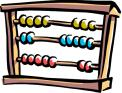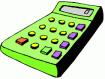A spreadsheet is a document which is divided into rows and columns to record such things as items in inventory, income and expenses, debits and credits.

Spreadsheets are designed to ease the management of numbers and calculations. They calculate totals, averages, percentages, budgets, and complex financial and scientific formulas.  This is a sample of a spreadsheet.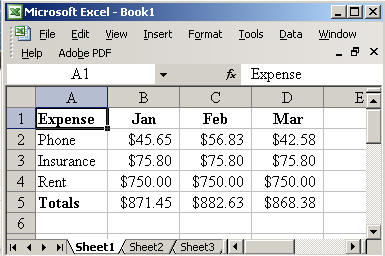• columns
• rows
• and their intersections are called cells

In a spreadsheet the COLUMN is defined as the vertical space that is going up and down the window. Letters are used to designate each COLUMN'S location.  The highlighted part of the spreadsheet is a column, and it is labeled B.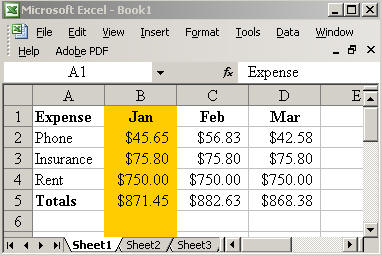In a spreadsheet the ROW is the horizontal space that is going across the window. Numbers are used to designate each ROW'S location.  The highlighted part of the spreadsheet is a row, and it is labeled 3.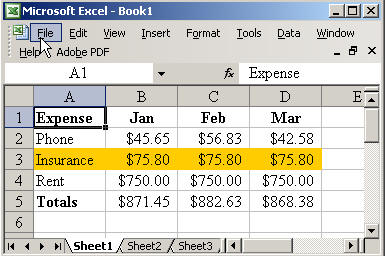In a spreadsheet the CELL is the space where a specified row and column intersect.  Each CELL is assigned a name according to its COLUMN letter and ROW number.  The highlighted part of the spreadsheet is a cell, and it is labeled C3.  When naming a cell, you should put the column first and the row second.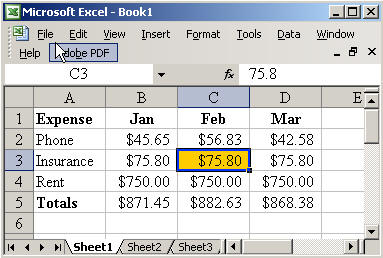In a spreadsheet there are three basic types of data that can be entered.

• labels - (text with no numerical value) Column A and Row 1 are labels because they contain text.  They help identify what we are talking about.

• values - (just numbers) Cells B2, B3, B4, C2, C3, C4, D2, D3, and D4 are values because they are numbers.  These values just happen to be  expressed in dollars, but you could enter any type of number here.

• formulas - (a mathematical equation used to calculate) Cells B5, C5, and D5 are formulas because they add together the value cells above them.  So the formula in B5 looks like this =SUM(B2:B4).  All formulas must begin with the = sign.  This formula tells the program to add together the values in cells B2, B3, and B4 and put the answer in B5.  Although this formula is a simple one, spreadsheets can do very sophisticated calculations.